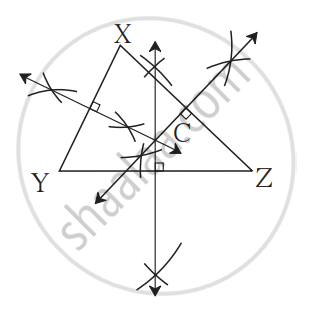# Perpendicular Bisectors of the Sides of an Acute-angled Triangle

## Notes

### Perpendicular Bisectors of the Sides of an acute-angled triangle:

1. Use a ruler to draw an acute-angled triangle.
2. Draw the perpendicular bisectors of each side of an acute-angled triangle.
3. The perpendicular bisectors of the sides of an acute-angled triangle are concurrent.
4. Name their point of concurrence ‘C’. Measure the distance between C and the vertices of the triangle. Note that CX = CY = CZ### Properties of Perpendicular Bisectors of the Sides of an acute-angled triangle:

• A triangle has 3 perpendicular bisectors.
• The 3 perpendicular bisectors of the triangle are concurrent.
• The point of concurrence i.e., C is called the circumcentre.
• In an acute-angled triangle, the circumcentre is in the interior of the triangle.
If you would like to contribute notes or other learning material, please submit them using the button below.

### Shaalaa.com

Construct Perpendicular Bisectors Of Acute-angled triangle [00:02:20]
S
0%